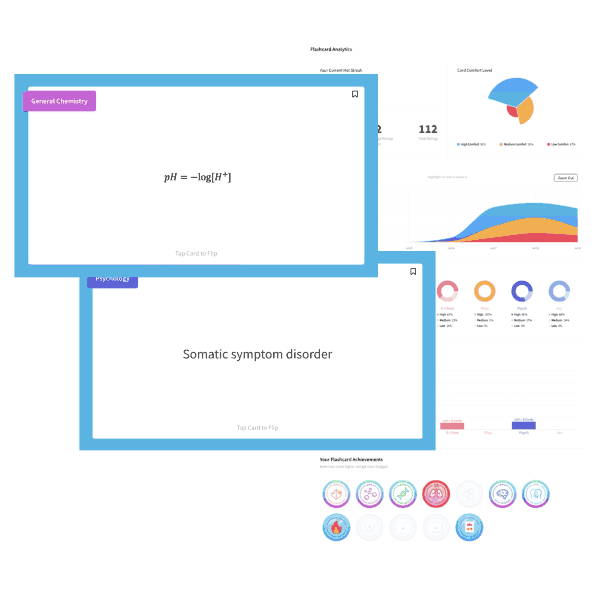# MCAT Biology Question — Predominate Structures

Glycine is an achiral amino acid with pKa values of 2.34 and 9.60. Which of the following is the predominant structure of glycine at a physiological pH?##### Click for Explanation

This questions asks the examinee to determine the predominate structure of the amino acid glycine. Given the pKa value for the amine and carboxylic acid group, you can apply the Henderson-Hasselbalch equation to figure out whether the substituent exists as an acid or conjugate base at a physiological pH of 7.4.

COOH:

pH = pKa + log[COO]/[COOH]

7.4 = 2.34 + log[COO]/[COOH]

5.06 = log[COO]/[COOH]

105.06 = [COO]/[COOH]

[COO] = 105.06[COOH]
Thus, the conjugate base COO= is the predominate form.

NH3+:

pH = pKa + log[NH2]/[NH3+]

7.4 = 9.60 + log[NH2]/[NH3+]

-2.2 = log[NH2]/[NH3+]

10-2.2 = [NH2]/[NH3+]

102.2[NH2] = [NH3+]
Thus, the acid NH3+ predominates.

Therefore, the structure in answer choice D is the most common form of glycine at a physiological pH.

## Want more MCAT practice?

### We’ve got options for every schedule and learning style!

From the best online MCAT course created by top instructors with 524+ MCAT scores to the most representative full-length practice exams and private tutoring, we can custom tailor your MCAT prep to your goals!

Not sure which option is right for you? Schedule a free MCAT consultation with an MCAT expert using the form below. No obligation, just expert advice.

Create your Free Account to access our MCAT Flashcards